# Synchronous generators

Feb. 11, 2017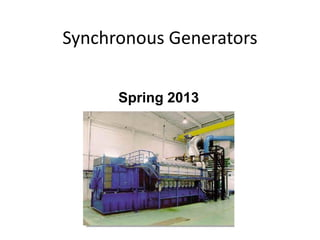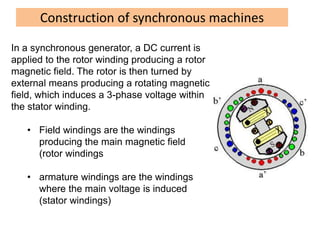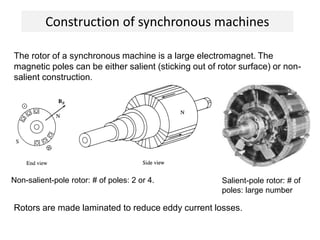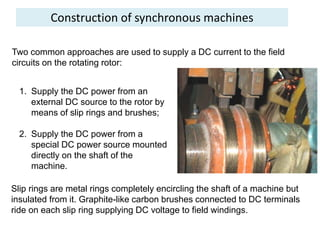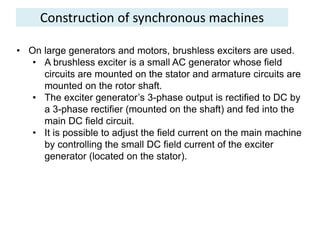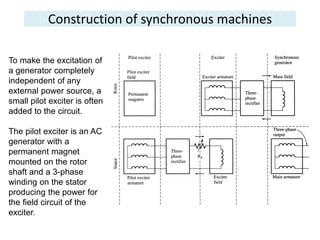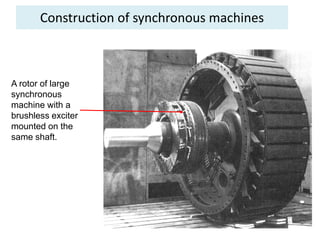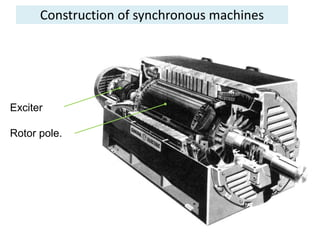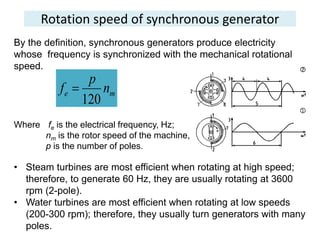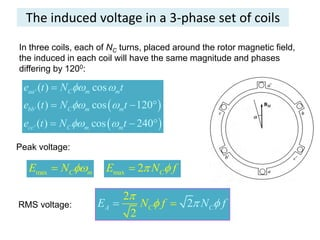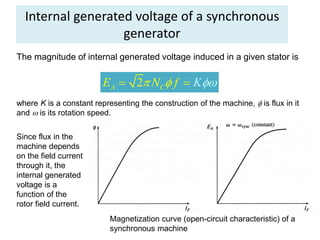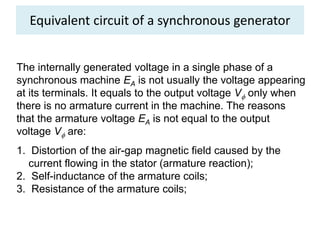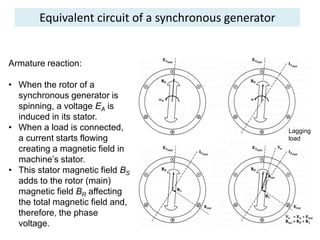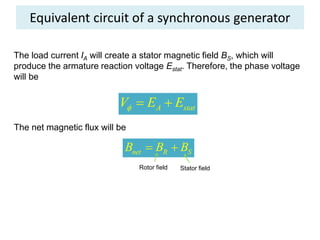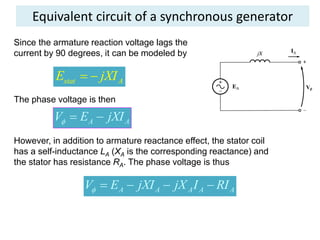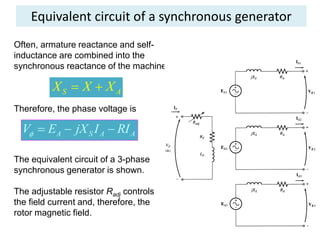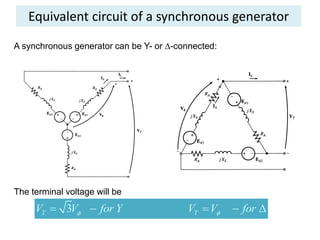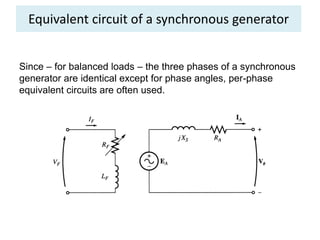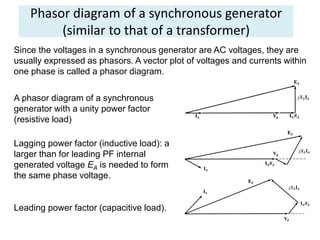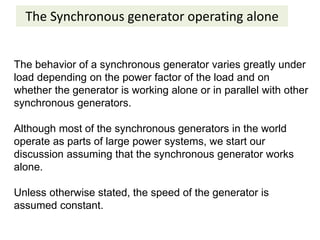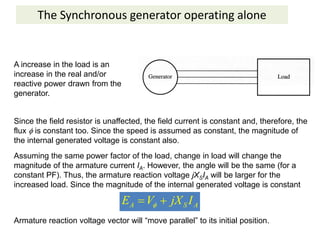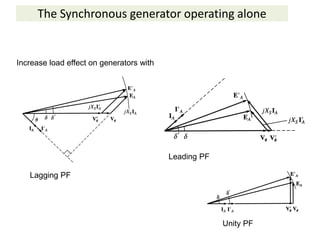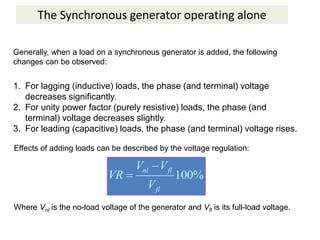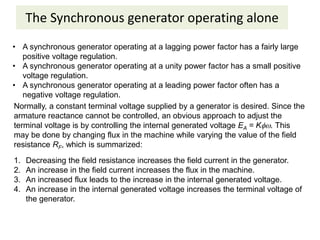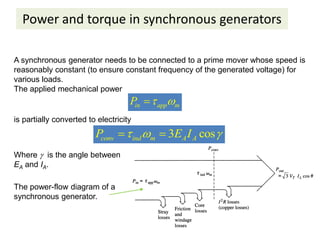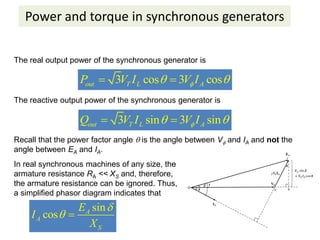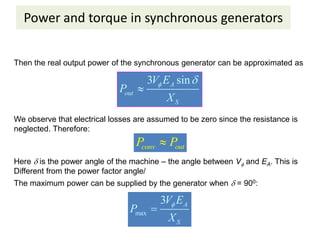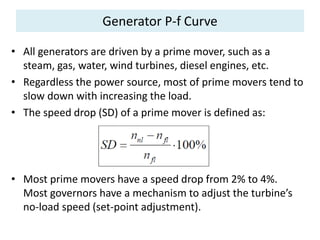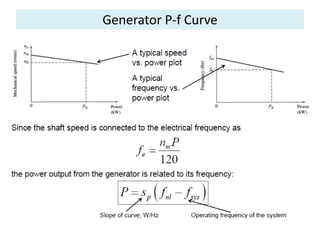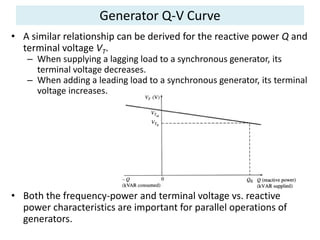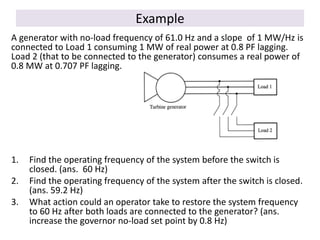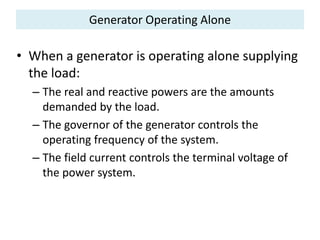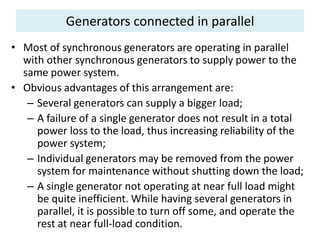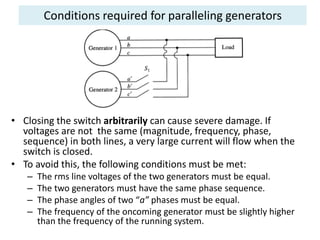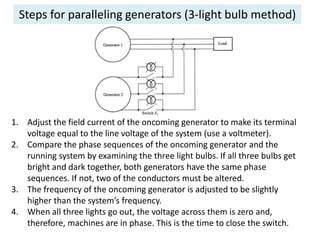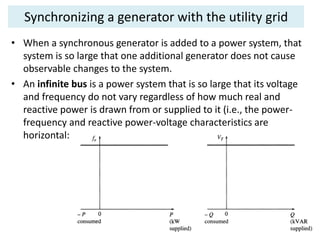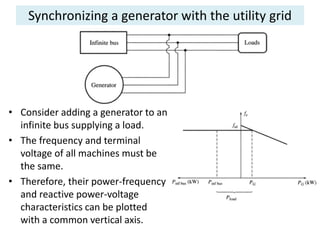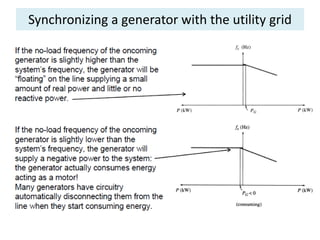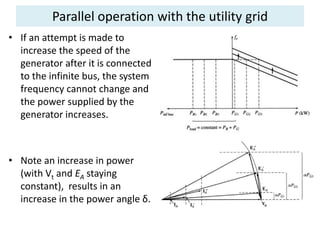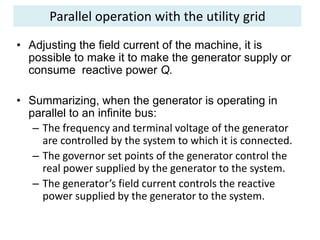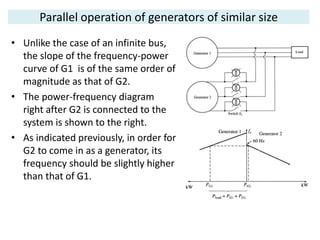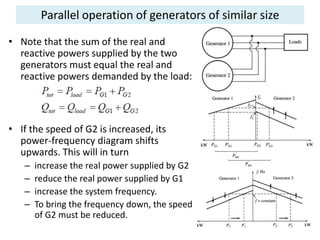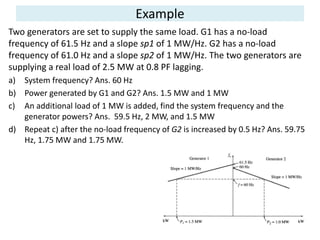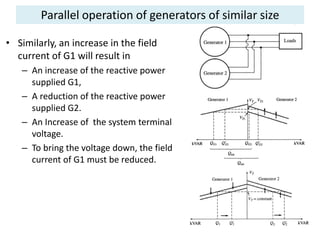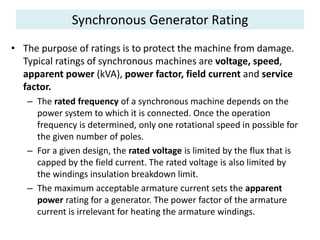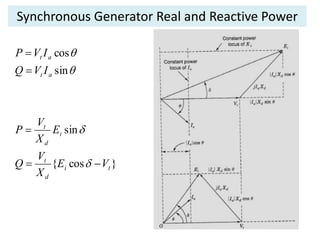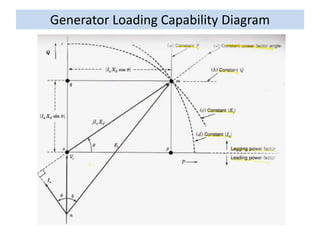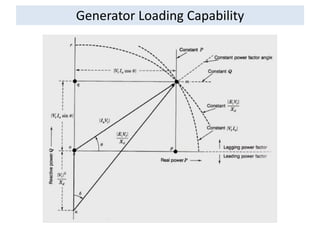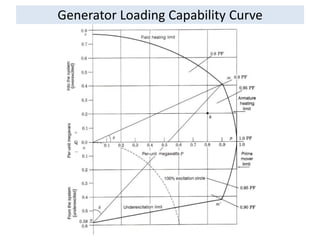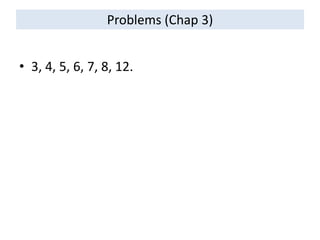1 of 50

### Synchronous generators

• 2. Construction of synchronous machines In a synchronous generator, a DC current is applied to the rotor winding producing a rotor magnetic field. The rotor is then turned by external means producing a rotating magnetic field, which induces a 3-phase voltage within the stator winding. • Field windings are the windings producing the main magnetic field (rotor windings • armature windings are the windings where the main voltage is induced (stator windings)
• 3. Construction of synchronous machines The rotor of a synchronous machine is a large electromagnet. The magnetic poles can be either salient (sticking out of rotor surface) or non- salient construction. Non-salient-pole rotor: # of poles: 2 or 4. Salient-pole rotor: # of poles: large number Rotors are made laminated to reduce eddy current losses.
• 4. Construction of synchronous machines Two common approaches are used to supply a DC current to the field circuits on the rotating rotor: 1. Supply the DC power from an external DC source to the rotor by means of slip rings and brushes; 2. Supply the DC power from a special DC power source mounted directly on the shaft of the machine. Slip rings are metal rings completely encircling the shaft of a machine but insulated from it. Graphite-like carbon brushes connected to DC terminals ride on each slip ring supplying DC voltage to field windings.
• 5. Construction of synchronous machines • On large generators and motors, brushless exciters are used. • A brushless exciter is a small AC generator whose field circuits are mounted on the stator and armature circuits are mounted on the rotor shaft. • The exciter generator’s 3-phase output is rectified to DC by a 3-phase rectifier (mounted on the shaft) and fed into the main DC field circuit. • It is possible to adjust the field current on the main machine by controlling the small DC field current of the exciter generator (located on the stator).
• 6. Construction of synchronous machines To make the excitation of a generator completely independent of any external power source, a small pilot exciter is often added to the circuit. The pilot exciter is an AC generator with a permanent magnet mounted on the rotor shaft and a 3-phase winding on the stator producing the power for the field circuit of the exciter.
• 7. Construction of synchronous machines A rotor of large synchronous machine with a brushless exciter mounted on the same shaft.
• 8. Construction of synchronous machines Exciter Rotor pole.
• 9. Rotation speed of synchronous generator By the definition, synchronous generators produce electricity whose frequency is synchronized with the mechanical rotational speed. Where fe is the electrical frequency, Hz; nm is the rotor speed of the machine, rpm; p is the number of poles. • Steam turbines are most efficient when rotating at high speed; therefore, to generate 60 Hz, they are usually rotating at 3600 rpm (2-pole). • Water turbines are most efficient when rotating at low speeds (200-300 rpm); therefore, they usually turn generators with many poles. me n p f 120 
• 10. The induced voltage in a 3-phase set of coils In three coils, each of NC turns, placed around the rotor magnetic field, the induced in each coil will have the same magnitude and phases differing by 1200:     ' ' ' ( ) cos ( ) cos 120 ( ) cos 240 aa C m m bb C m m cc C m m e t N t e t N t e t N t              Peak voltage: max C mE N  max 2 CE N f  RMS voltage: 2 2 2CA CNE Nf f    
• 11. Internal generated voltage of a synchronous generator The magnitude of internal generated voltage induced in a given stator is 2A CE N f K    where K is a constant representing the construction of the machine,  is flux in it and  is its rotation speed. Since flux in the machine depends on the field current through it, the internal generated voltage is a function of the rotor field current. Magnetization curve (open-circuit characteristic) of a synchronous machine
• 12. Equivalent circuit of a synchronous generator The internally generated voltage in a single phase of a synchronous machine EA is not usually the voltage appearing at its terminals. It equals to the output voltage V only when there is no armature current in the machine. The reasons that the armature voltage EA is not equal to the output voltage V are: 1. Distortion of the air-gap magnetic field caused by the current flowing in the stator (armature reaction); 2. Self-inductance of the armature coils; 3. Resistance of the armature coils;
• 13. Equivalent circuit of a synchronous generator Armature reaction: • When the rotor of a synchronous generator is spinning, a voltage EA is induced in its stator. • When a load is connected, a current starts flowing creating a magnetic field in machine’s stator. • This stator magnetic field BS adds to the rotor (main) magnetic field BR affecting the total magnetic field and, therefore, the phase voltage. Lagging load
• 14. Equivalent circuit of a synchronous generator The load current IA will create a stator magnetic field BS, which will produce the armature reaction voltage Estat. Therefore, the phase voltage will be A statV E E   The net magnetic flux will be net R SB B B  Rotor field Stator field
• 15. Equivalent circuit of a synchronous generator Since the armature reaction voltage lags the current by 90 degrees, it can be modeled by stat AE jXI  The phase voltage is then A AV E jXI   However, in addition to armature reactance effect, the stator coil has a self-inductance LA (XA is the corresponding reactance) and the stator has resistance RA. The phase voltage is thus A A A A AV E jXI jX I RI    
• 16. Equivalent circuit of a synchronous generator Often, armature reactance and self- inductance are combined into the synchronous reactance of the machine: S AX X X  A S A AV E jX I RI    Therefore, the phase voltage is The equivalent circuit of a 3-phase synchronous generator is shown. The adjustable resistor Radj controls the field current and, therefore, the rotor magnetic field.
• 17. Equivalent circuit of a synchronous generator A synchronous generator can be Y- or -connected: The terminal voltage will be 3T TV V for Y V V for       
• 18. Equivalent circuit of a synchronous generator Since – for balanced loads – the three phases of a synchronous generator are identical except for phase angles, per-phase equivalent circuits are often used.
• 19. Phasor diagram of a synchronous generator (similar to that of a transformer) Since the voltages in a synchronous generator are AC voltages, they are usually expressed as phasors. A vector plot of voltages and currents within one phase is called a phasor diagram. A phasor diagram of a synchronous generator with a unity power factor (resistive load) Lagging power factor (inductive load): a larger than for leading PF internal generated voltage EA is needed to form the same phase voltage. Leading power factor (capacitive load).
• 20. The Synchronous generator operating alone The behavior of a synchronous generator varies greatly under load depending on the power factor of the load and on whether the generator is working alone or in parallel with other synchronous generators. Although most of the synchronous generators in the world operate as parts of large power systems, we start our discussion assuming that the synchronous generator works alone. Unless otherwise stated, the speed of the generator is assumed constant.
• 21. The Synchronous generator operating alone Effects of load changesA increase in the load is an increase in the real and/or reactive power drawn from the generator. Since the field resistor is unaffected, the field current is constant and, therefore, the flux  is constant too. Since the speed is assumed as constant, the magnitude of the internal generated voltage is constant also. Assuming the same power factor of the load, change in load will change the magnitude of the armature current IA. However, the angle will be the same (for a constant PF). Thus, the armature reaction voltage jXSIA will be larger for the increased load. Since the magnitude of the internal generated voltage is constant A S AE V jX I  Armature reaction voltage vector will “move parallel” to its initial position.
• 22. The Synchronous generator operating alone Increase load effect on generators with Lagging PF Leading PF Unity PF
• 23. The Synchronous generator operating alone 1. For lagging (inductive) loads, the phase (and terminal) voltage decreases significantly. 2. For unity power factor (purely resistive) loads, the phase (and terminal) voltage decreases slightly. 3. For leading (capacitive) loads, the phase (and terminal) voltage rises. Generally, when a load on a synchronous generator is added, the following changes can be observed: Effects of adding loads can be described by the voltage regulation: 100% nl fl fl V V VR V   Where Vnl is the no-load voltage of the generator and Vfl is its full-load voltage.
• 24. The Synchronous generator operating alone • A synchronous generator operating at a lagging power factor has a fairly large positive voltage regulation. • A synchronous generator operating at a unity power factor has a small positive voltage regulation. • A synchronous generator operating at a leading power factor often has a negative voltage regulation. Normally, a constant terminal voltage supplied by a generator is desired. Since the armature reactance cannot be controlled, an obvious approach to adjust the terminal voltage is by controlling the internal generated voltage EA = K. This may be done by changing flux in the machine while varying the value of the field resistance RF, which is summarized: 1. Decreasing the field resistance increases the field current in the generator. 2. An increase in the field current increases the flux in the machine. 3. An increased flux leads to the increase in the internal generated voltage. 4. An increase in the internal generated voltage increases the terminal voltage of the generator.
• 25. Power and torque in synchronous generators A synchronous generator needs to be connected to a prime mover whose speed is reasonably constant (to ensure constant frequency of the generated voltage) for various loads. The applied mechanical power in app mP   is partially converted to electricity 3 cosconv ind m A AP E I    Where  is the angle between EA and IA. The power-flow diagram of a synchronous generator.
• 26. Power and torque in synchronous generators The real output power of the synchronous generator is 3 cos 3 cosout T L AP V I V I   The reactive output power of the synchronous generator is 3 sin 3 sinout T L AQ V I V I   Recall that the power factor angle  is the angle between V and IA and not the angle between EA and IA. In real synchronous machines of any size, the armature resistance RA << XS and, therefore, the armature resistance can be ignored. Thus, a simplified phasor diagram indicates that sin cos A A S E I X   
• 27. Power and torque in synchronous generators Then the real output power of the synchronous generator can be approximated as 3 sinA out S V E P X    We observe that electrical losses are assumed to be zero since the resistance is neglected. Therefore: conv outP P Here  is the power angle of the machine – the angle between V and EA. This is Different from the power factor angle/ The maximum power can be supplied by the generator when  = 900: max 3 A S V E P X  
• 28. Generator P-f Curve • All generators are driven by a prime mover, such as a steam, gas, water, wind turbines, diesel engines, etc. • Regardless the power source, most of prime movers tend to slow down with increasing the load. • The speed drop (SD) of a prime mover is defined as: • Most prime movers have a speed drop from 2% to 4%. Most governors have a mechanism to adjust the turbine’s no-load speed (set-point adjustment).
• 30. Generator Q-V Curve • A similar relationship can be derived for the reactive power Q and terminal voltage VT. – When supplying a lagging load to a synchronous generator, its terminal voltage decreases. – When adding a leading load to a synchronous generator, its terminal voltage increases. • Both the frequency-power and terminal voltage vs. reactive power characteristics are important for parallel operations of generators.
• 31. Example A generator with no-load frequency of 61.0 Hz and a slope of 1 MW/Hz is connected to Load 1 consuming 1 MW of real power at 0.8 PF lagging. Load 2 (that to be connected to the generator) consumes a real power of 0.8 MW at 0.707 PF lagging. 1. Find the operating frequency of the system before the switch is closed. (ans. 60 Hz) 2. Find the operating frequency of the system after the switch is closed. (ans. 59.2 Hz) 3. What action could an operator take to restore the system frequency to 60 Hz after both loads are connected to the generator? (ans. increase the governor no-load set point by 0.8 Hz)
• 32. Generator Operating Alone • When a generator is operating alone supplying the load: – The real and reactive powers are the amounts demanded by the load. – The governor of the generator controls the operating frequency of the system. – The field current controls the terminal voltage of the power system.
• 33. Generators connected in parallel • Most of synchronous generators are operating in parallel with other synchronous generators to supply power to the same power system. • Obvious advantages of this arrangement are: – Several generators can supply a bigger load; – A failure of a single generator does not result in a total power loss to the load, thus increasing reliability of the power system; – Individual generators may be removed from the power system for maintenance without shutting down the load; – A single generator not operating at near full load might be quite inefficient. While having several generators in parallel, it is possible to turn off some, and operate the rest at near full-load condition.
• 34. Conditions required for paralleling generators • Closing the switch arbitrarily can cause severe damage. If voltages are not the same (magnitude, frequency, phase, sequence) in both lines, a very large current will flow when the switch is closed. • To avoid this, the following conditions must be met: – The rms line voltages of the two generators must be equal. – The two generators must have the same phase sequence. – The phase angles of two “a” phases must be equal. – The frequency of the oncoming generator must be slightly higher than the frequency of the running system.
• 35. Steps for paralleling generators (3-light bulb method) 1. Adjust the field current of the oncoming generator to make its terminal voltage equal to the line voltage of the system (use a voltmeter). 2. Compare the phase sequences of the oncoming generator and the running system by examining the three light bulbs. If all three bulbs get bright and dark together, both generators have the same phase sequences. If not, two of the conductors must be altered. 3. The frequency of the oncoming generator is adjusted to be slightly higher than the system’s frequency. 4. When all three lights go out, the voltage across them is zero and, therefore, machines are in phase. This is the time to close the switch.
• 36. Synchronizing a generator with the utility grid • When a synchronous generator is added to a power system, that system is so large that one additional generator does not cause observable changes to the system. • An infinite bus is a power system that is so large that its voltage and frequency do not vary regardless of how much real and reactive power is drawn from or supplied to it (i.e., the power- frequency and reactive power-voltage characteristics are horizontal:
• 37. Synchronizing a generator with the utility grid • Consider adding a generator to an infinite bus supplying a load. • The frequency and terminal voltage of all machines must be the same. • Therefore, their power-frequency and reactive power-voltage characteristics can be plotted with a common vertical axis.
• 38. Synchronizing a generator with the utility grid
• 39. Parallel operation with the utility grid • If an attempt is made to increase the speed of the generator after it is connected to the infinite bus, the system frequency cannot change and the power supplied by the generator increases. • Note an increase in power (with Vt and EA staying constant), results in an increase in the power angle δ.
• 40. Parallel operation with the utility grid • Adjusting the field current of the machine, it is possible to make it to make the generator supply or consume reactive power Q. • Summarizing, when the generator is operating in parallel to an infinite bus: – The frequency and terminal voltage of the generator are controlled by the system to which it is connected. – The governor set points of the generator control the real power supplied by the generator to the system. – The generator’s field current controls the reactive power supplied by the generator to the system.
• 41. Parallel operation of generators of similar size • Unlike the case of an infinite bus, the slope of the frequency-power curve of G1 is of the same order of magnitude as that of G2. • The power-frequency diagram right after G2 is connected to the system is shown to the right. • As indicated previously, in order for G2 to come in as a generator, its frequency should be slightly higher than that of G1.
• 42. Parallel operation of generators of similar size • Note that the sum of the real and reactive powers supplied by the two generators must equal the real and reactive powers demanded by the load: • If the speed of G2 is increased, its power-frequency diagram shifts upwards. This will in turn – increase the real power supplied by G2 – reduce the real power supplied by G1 – increase the system frequency. – To bring the frequency down, the speed of G2 must be reduced.
• 43. Example Two generators are set to supply the same load. G1 has a no-load frequency of 61.5 Hz and a slope sp1 of 1 MW/Hz. G2 has a no-load frequency of 61.0 Hz and a slope sp2 of 1 MW/Hz. The two generators are supplying a real load of 2.5 MW at 0.8 PF lagging. a) System frequency? Ans. 60 Hz b) Power generated by G1 and G2? Ans. 1.5 MW and 1 MW c) An additional load of 1 MW is added, find the system frequency and the generator powers? Ans. 59.5 Hz, 2 MW, and 1.5 MW d) Repeat c) after the no-load frequency of G2 is increased by 0.5 Hz? Ans. 59.75 Hz, 1.75 MW and 1.75 MW.
• 44. Parallel operation of generators of similar size • Similarly, an increase in the field current of G1 will result in – An increase of the reactive power supplied G1, – A reduction of the reactive power supplied G2. – An Increase of the system terminal voltage. – To bring the voltage down, the field current of G1 must be reduced.
• 45. Synchronous Generator Rating • The purpose of ratings is to protect the machine from damage. Typical ratings of synchronous machines are voltage, speed, apparent power (kVA), power factor, field current and service factor. – The rated frequency of a synchronous machine depends on the power system to which it is connected. Once the operation frequency is determined, only one rotational speed in possible for the given number of poles. – For a given design, the rated voltage is limited by the flux that is capped by the field current. The rated voltage is also limited by the windings insulation breakdown limit. – The maximum acceptable armature current sets the apparent power rating for a generator. The power factor of the armature current is irrelevant for heating the armature windings.
• 46. Synchronous Generator Real and Reactive Power }cos{ sin sin cos ti d t i d t at at VE X V Q E X V P IVQ IVP        
• 50. Problems (Chap 3) • 3, 4, 5, 6, 7, 8, 12.